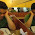As of May 4 2007 the scripts will autodetect your timezone settings. Nothing here has to be changed, but there are a few things

## Saturday, May 14, 2011

### Sum of a list in Mathematica

Problem: We want to calculate the sum of the elements of a list of numbers. Suppose this list is named l and has been assigned the value {1,2, ..., 20}

Method 1: ( Use the Mathematica function to total the values in a list. )
Total[l]

Method 2: ( Use the formula for the sum of an arithmetic sequence, with l[] being the first element, l[[Length[l]]] being the last element and Length[l] the number of elements.)
(l[] + l[[Length[l]]])*Length[l]/2

Method 3: ( Go through all the elements from the first to the last and add them to a running total. )
Sum[l[[k]], {k, 1, Length[l]}]

Method 4: ( Recursively define the total of a list. )
s[{}] := 0
s[l_] := First[l] + s[Rest[l]]

Method 5: ( Use some intricate method of the Mathematica language. )
Fold[#1 + #2 &, 0, l]

All five methods give the same result 210.

Set l := Array[#&,20];

Or use the following to test the commands.

Total[Array[#&,20]]

(Array[#&,20][] + Array[#&,20][[Length[Array[#&,20]]]])*Length[Array[#&,20]]/2

Sum[Array[#&,20][[k]], {k, 1, Length[Array[#&,20]]}]

s[{}] := 0
s[l_] := First[l] + s[Rest[l]]
s[Array[#&,20]]

Fold[#1 + #2 &, 0, Array[#&,20]]

1.I have a list, how can i sum it using sumation? I didn't understand with your example. This is what i'm trying to do.

r := RandomReal[{-10, 10}];
s = {};
x = 1; While[x <= 1000, AppendTo[s, r]; x++]
ListLinePlot[s]
Total[s]
c = Count[s, _]
Take[s, 1]

2.You are not building a list.
You should have done s = AppendTo[s, r]

Go to http://stackoverflow.com/ for expert answers on Mathematica and other computer languages.

3.trying to work my way around this but makes my head spin...urrgh. But thumbs up for the detailed explanation though. Think I will head over to Wollfram Alpha :)

## Welcome to The Bridge

Mathematics: is it the fabric of MEST?
This is my voyage
My continuous mission
To uncover hidden structures
To create new theorems and proofs
To boldly go where no man has gone before(Raumpatrouille – Die phantastischen Abenteuer des Raumschiffes Orion, colloquially aka Raumpatrouille Orion was the first German science fiction television series. Its seven episodes were broadcast by ARD beginning September 17, 1966. The series has since acquired cult status in Germany. Broadcast six years before Star Trek first aired in West Germany (in 1972), it became a huge success.)